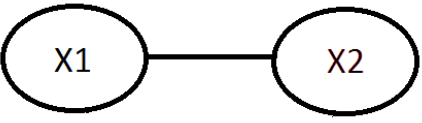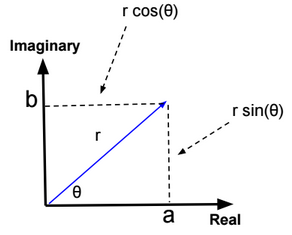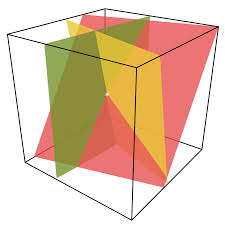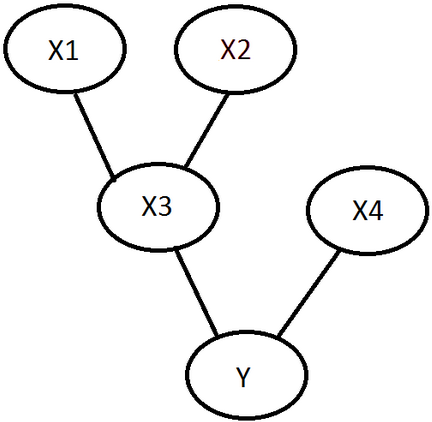# Basic Statistics

Basic statistics for a discrete variable X:

Mean(μ) = Expect Value E[X]

=$$\frac{1}{n} \sum_{i=1}^{n} x_{i}$$

Median

If n is odd then $$x_{\frac{n+1}{2}}$$ else $$\frac{x_{\frac{n}{2}} + x_{\frac{n+2}{2}}}{2}$$

Variance ($$\sigma^{2}=E_{x \sim p(x)}[(X-E[X])^2]$$)

(n-1 is called degree of freedom)

=$$\frac{1}{n-1}\sum_{i=1}^{n} (x_{i} -\mu)^{2}$$

Standard deviation ($$\sigma$$)

=$$\sqrt{\sigma^{2}}$$

Mode

is the value x at which its probability mass function takes its maximum value. For example the mode of {1,1,1,2,2,3,4} is 1 because it appears 3 times

Covariance(X,Y)

$$= E[(X-E[X])(Y-E[Y])]$$ $$= E[XY] -E[X]E[Y]$$ $$= \frac{1}{n-1} \sum_{i=1}^{n} (X_i – μ_X)(Y_i – μ_Y)$$

Correlation(X,Y)

#### =$$\frac{Covariance(X,Y)}{\sqrt{Var(X).Var(Y)}}$$

Standard error

$$= \frac{σ}{\sqrt{n}}$$

Basic statistics for a continuous variable X:

 Mean(μ) = Expect Value E[X] =$$\int_{all\, x} p(x)\,x\,dx$$ Median m such as p(x<=m) = .5 Variance ($$\sigma^{2}=E_{x \sim p(x)}[(X-E[X])^2]$$) =$$\int_{all\, x} p(x)\,(x -\mu)^{2}\,dx$$ Standard deviation ($$\sigma$$) =$$\sqrt{\sigma^{2}}$$ Mode is the value x at which its probability density function has its maximum value

Examples:

For a set {-1, -1, 1, 1} => Mean = 0, Variance = 1.33, Standard deviation = 1.15

If $$x \in [0, +\infty]$$ and p(x) = exp(-x) => Mean = 1, Variance = 1, Standard deviation = 1

Expected value

Expectations are linear. E[X+Y] = E[X] + E[Y]

# Probability Distributions

A random variable is a set of outcomes from a random experiment.

A probability distribution is a function that returns the probability of occurrence of an outcome. For discrete random variables, this function is called “Probability Mass Function”. For continuous variables, this function is called “Probability Density Function”.

A joint probability distribution is a function that returns the probability of joint occurrence of outcomes from two or more random variables. If random variables are independent then the joint probability distribution is equal to the product of the probability distribution of each random variable.

A conditional probability distribution is the probability distribution of a random variable given another random variables.

Example:

 X P(X) A 0.2 B 0.8

P(X) is the probability distribution of X.

 Y P(Y) C 0.1 D 0.9

P(Y) is the probability distribution of Y.

 X Y P(X,Y) A C 0.1 A D 0.1 B D 0.8

P(X,Y) is the joint probability distribution of X and Y.

 X P(X|Y=D) = P(X,Y)/P(Y=D) A 0.1/0.9 B 0.8/0.9

P(X|Y=D) is the conditional probability distribution of X given Y = D.

Marginal probability

Sometimes we know the probability distribution over a set of variables and we want to know the probability distribution over just a subset of them. The probability distribution over the subset is known as the marginal probability distribution.

For example, suppose we have discrete random variables x and y, and we know P(x, y). We can find P(x) with the sum rule: $$P(x) = \sum_y P(x,y)$$

Below the statistical properties of some distributions.

Binomial distribution

Nb of output values = 2 (like coins)

n = number of trials

p = probability of success

P(X) = $$C_n^X * p^{X} * (1-p)^{n-X}$$

Expected value = n.p

Variance = n.p.(1-p)

Example:

If we flip a fair coin (p=0.5) three time (n=3), what’s the probability of getting two heads and one tail?

P(X=2) = P(2H and 1T) = P(HHT + HTH + THH) = P(HHT) + P(HTH) + P(THH) = p.p(1-p) + p.(1-p).p + (1-p).p.p = $$C_3^2.p^2.(1-p)$$

Bernoulli distribution

Bernoulli distribution is a special case of the binomial distribution with n=1.

Nb of output values = 2 (like coins)

n = 1 (number of trials)

p = probability of success

X $$\in$$ {0,1}

P(X) = $$p^{X} * (1-p)^{1-X}$$

Expected value = p

Variance = p.(1-p)

Example:

If we flip a fair coin (p=0.5) one time (n=1), what’s the probability of getting 0 head?

P(X=0) = P(0H) = P(1T) = 1-p = $$p^0.(1-p)^1$$

Multinomial distribution

It’s a generalization of Binomial distribution. In a Multinomial distribution we can have more than two outcomes. For each outcome, we can assign a probability of success.

Normal (Gaussian) distribution

P(x) = $$\frac{1}{\sigma \sqrt{2\pi}} exp(-\frac{1}{2} (\frac{x-\mu}{\sigma})^{2})$$

σ and μ are sufficient statistics (sufficient to describe the whole curve)

Expected value = $$\int_{-\infty}^{+\infty} p(x) x \, dx$$

Variance = $$\int_{-\infty}^{+\infty} (x – \mu)^2 p(x) \, dx$$

Standard Normal distribution (Z-Distribution)

It’s a normal distribution with mean = 0 and standard deviation = 1.

P(z) = $$\frac{1}{\sqrt{2\pi}} exp(-\frac{1}{2} z^2)$$

Cumulative distribution function:

$$P(x \leq z) = \int_{-\infty}^{z} \frac{1}{\sqrt{2\pi}} exp(-\frac{1}{2} x^2) \, dx$$

Exponential Family distribution

$$P(x;\theta) = h(x)\exp \left(\eta (θ)\cdot T(x)-A(θ)\right)$$, where T(x), h(x), η(θ), and A(θ) are known functions.

θ = vector of parameters.

T(x) = vector of “sufficient statistics”.

A(θ) = cumulant generating function.

The Binomial distribution is an Exponential Family distribution

$$P(x)=C_n^x\ p^{x}(1-p)^{n-x},\quad x\in \{0,1,2,\ldots ,n\}$$

This can equivalently be written as:

$$P(x)=C_n^x\ exp (log(\frac{p}{1-p}).x – (-n.log(1-p)))$$

The Normal distribution is an Exponential Family distribution

Consider a random variable distributed normally with mean μ and variance $$σ^2$$. The probability density function could be written as: $$P(x;θ) = h(x)\exp(η(θ).T(x)-A(θ))$$

With:

$$h(x)={\frac{1}{\sqrt {2\pi \sigma ^{2}}}}exp^{-{\frac {x^{2}}{2\sigma ^{2}}}}$$ $$T(x)={\frac {x}{\sigma }}$$ $$A(\mu)={\frac {\mu ^{2}}{2\sigma ^{2}}}$$ $$\eta(\mu)={\frac {\mu }{\sigma }}$$

Poisson distribution

The Poisson distribution is popular for modelling the number of times an event occurs in an interval of time or space (eg. number of arrests, number of fish in a trap…).

In a Poisson distribution, values are discrete and can’t be negative.

The probability mass function is defined as: $$P(x=k)=\frac{λ^k.e^{-λ}}{k!}$$, k is the number of occurrences. λ is the expected number of occurrences.

Exponential distribution

The exponential distribution has a probability distribution with a sharp point at x = 0.

$$P(x; λ) = λ.1_{x≥0}.exp (−λx)$$

Laplace distribution

Laplace distribution has a sharp peak of probability mass at an arbitrary point μ. The probability mass function is defined as $$Laplace(x;μ,γ) = \frac{1}{2γ} exp(-\frac{|x-μ|}{γ})$$

Laplace distribution is a distribution that is symmetrical and more “peaky” than a normal distribution. The dispersion of the data around the mean is higher than that of a normal distribution. Laplace distribution is also sometimes called the double exponential distribution.

Dirac distribution

The probability mass function is defined as $$P(x;μ) = δ(x).(x-μ)$$ such as δ(x) = 0 when x ≠ μ and $$\int_{-∞}^{∞} δ(x).(x-μ) dx= 1$$.

Empirical Distribution

Other known Exponential Family distributions

Dirichlet.

# Laplace Smoothing

Given a set S={a1, a1, a1, a2}.

Laplace smoothed estimate for P(x) with domain of x in {a1, a2, a3}:

$$P(x=a1)=\frac{3 + 1}{4 + 3}$$ $$P(x=a2)=\frac{1 + 1}{4 + 3}$$ $$P(x=a3)=\frac{0 + 1}{4 + 3}$$

# Maximum Likelihood Estimation

Given three independent data points $$x_1=1, x_2=0.5, x_3=1,5$$, what the mean μ of a normal distribution that these three points are more likely to come from (we suppose the variance=1).

If μ = 4, then the probabilities $$P(X=x_1), P(X=x_2), P(X=x_3)$$ will be low, and $$P(x_1,x_2,x_3) = P(X=x_1)*P(X=x_2)*P(X=x_3)$$ will be also low.

If μ = 1, then the probabilities $$P(X=x_1), P(X=x_2), P(X=x_3)$$ will be high, and $$P(x_1,x_2,x_3) = P(X=x_1)*P(X=x_2)*P(X=x_3)$$ will be also high. Which means that the three points are more likely to come from a normal distribution with mean μ = 1.

The likelihood function is defined as: $$P(x_1,x_2,x_3; μ)$$

# Central Limit Theorem

The central limit theorem states that if you have a population with mean μ and standard deviation σ and take sufficiently large random samples from the population with replacement , then the distribution of sample means will be approximately normally distributed.

# Bayesian Network

X1, X2 are random variables.P(X1,X2) = P(X2,X1) = P(X2|X1) * P(X1) = P(X1|X2) * P(X2)

P(X1) is called prior probability.

P(X1|X2) is called posterior probability.

Example:

A mixed school having 60% boys and 40% girls as students. The girls wear trousers or skirts in equal numbers; the boys all wear trousers. An observer sees from a distance a student wearing a trouser. What is the probability this student is a girl?

The prior probability P(Girl): 0.4

The posterior probability P(Girl|Trouser): $$\frac{P(Trouser|Girl)*P(Girl)}{P(Trouser|Girl) * P(Girl) + P(Trouser|Boy) * P(Boy)} = 0.25$$

# Parameters estimation – Bayesian Approach Vs Frequentist Approach

There are two approaches that can be used to estimate the parameters of a model.

 Frequentist approach Bayesian approach $$arg\ \underset{θ}{max} \prod_{i=1}^m P(y^{(i)}|x^{(i)};θ)$$ $$arg\ \underset{θ}{max} P(θ|\{(x^{(i)}, y^{(i)})\}_{i=1}^m)$$ $$=arg\ \underset{θ}{max} \frac{P(\{(x^{(i)}, y^{(i)})\}_{i=1}^m|θ) * P(θ)}{P(\{(x^{(i)}, y^{(i)})\}_{i=1}^m)}$$ $$=arg\ \underset{θ}{max} P(\{(x^{(i)}, y^{(i)})\}_{i=1}^m|θ) * P(θ)$$ If $$\{(x^{(i)}, y^{(i)})\}$$ are independent, then: $$=arg\ \underset{θ}{max} \prod_{i=1}^m P((y^{(i)},x^{(i)})|θ) * P(θ)$$ To calculate P(θ) (called the prior), we assume that θ is Gaussian with mean 0 and variance $$\sigma^2$$. $$=arg\ \underset{θ}{max} log(\prod_{i=1}^m P((y^{(i)},x^{(i)})|θ) * P(θ))$$ $$=arg\ \underset{θ}{max} log(\prod_{i=1}^m P((y^{(i)},x^{(i)})|θ)) + log(P(θ))$$ after some few derivations, we will find the the expression is equivalent to the L2 regularized linear cost function. $$=arg\ \underset{θ}{min} \frac{1}{2} \sum_{i=1}^{n} (y^{(i)}-h_{θ}(x^{(i)}))^{2} + λ θ^Tθ$$

Because of the prior, Bayesian algorithms are less susceptible to overfitting.

Cumulative distribution function (CDF)

Given a random continuous variable S with density function p(s). The Cumulative distribution function $$F(s) = p(S<=s) = \int_{-∞}^{s} p(s) ds$$

F'(s) = p(s)

# Linear Algebra

A matrix is a 2-D array of numbers.

A tensor is a n-D array of numbers.

Matrices are associative but not commutative.

Not all square matrices have inverse. A matrix that is not invertible is called Singular or Degenerative.

A matrix is “singular” if any of the following are true:

-Any row or column contains all zeros.

-Any two rows or columns are identical.

-Any row or column is a linear combination of other rows or columns.

A square matrix A is singular, if and only if the determinant(A) = 0

Inner product of two vectors $$\overrightarrow{u}$$ and $$\overrightarrow{v}$$:

$$\overrightarrow{u} * \overrightarrow{v} = p * ||\overrightarrow{u}|| = p * \sqrt{\sum_{i=1}^{n}u_{i}^{2}} = u^{T} * v = \sum_{i=1}^{n}u_{i} * v_{i}$$

p is the projection of $$\overrightarrow{v}$$ on $$\overrightarrow{u}$$ and $$||\overrightarrow{u}||$$ is the Euclidean norm of $$\overrightarrow{u}$$.

$$A = \begin{bmatrix} a & b & c\\ d & e & f\\ g & h & i \end{bmatrix}$$

Determinant(A) = |A| = a(ei-hf) – b(di-gf) + c(dh – ge)

If $$f: R^{n*m} \mapsto R$$

$$\frac{\partial}{\partial A} f(A) = \begin{bmatrix} \frac{\partial}{\partial a_{11}}f(A) & … & \frac{\partial}{\partial a_{1m}} f(A)\\ … & … & …\\ \frac{\partial}{\partial a_{n1}}f(A) & … & \frac{\partial}{\partial a_{nm}}A\\ \end{bmatrix}$$

Example:

$$f(A) = a_{11} + … + a_{nm}$$

$$\frac{\partial}{\partial A} f(A) = \begin{bmatrix} 1 & … & 1\\ … & … & …\\ 1 & … & 1 \\ \end{bmatrix}$$

If A is a squared matrix:

trace(A) = $$\sum_{i=1}^n A_{ii}$$

trace(AB) = trace(BA)

trace(ABC) = trace(CAB) = trace(BCA)

trace(B) = trace($$B^T$$)

trace(a) = a

$$\frac{\partial}{\partial A} trace(AB) = B^T$$ $$\frac{\partial}{\partial A} trace(ABA^TC) = CAB + C^TAB^T$$

Eigenvector

Given a matrix A, if a vector μ satisfies the equation A*μ = λ*μ then μ is called an eigenvector for the matrix A, and λ is called the eigenvalue for the matrix A. The principal eigenvector for the matrix A is the eigenvalue with the largest eigenvalue.

Example:

The normalized eigenvectors for $$\begin{bmatrix}0 & 1 \\1 & 0 \end{bmatrix}$$ are $$\begin{bmatrix}\frac{1}{\sqrt{2}} \\ \frac{1}{\sqrt{2}} \end{bmatrix}$$ and $$\begin{bmatrix}-\frac{1}{\sqrt{2}} \\ \frac{1}{\sqrt{2}} \end{bmatrix}$$, the eigenvalues are 1 and -1.

Eigendecomposition

Given a squared matrix A∈$$R^{n*n}$$, ∃ Q∈$$R^{n*n}$$, Λ∈$$R^{n*n}$$ and Λ diagonal, such as $$A=QΛQ^T$$.

Q’s columns are the eigenvectors of $$A$$

Λ is the diagonal matrix whose diagonal elements are the eigenvalues

Example:

The eigendecomposition of $$\begin{bmatrix}0 & 1 \\1 & 0 \end{bmatrix}$$ is Q=$$\begin{bmatrix}\frac{1}{\sqrt{2}} & -\frac{1}{\sqrt{2}} \\ \frac{1}{\sqrt{2}} & \frac{1}{\sqrt{2}}\end{bmatrix}$$, Λ=$$\begin{bmatrix}\frac{1}{\sqrt{2}} & \frac{1}{\sqrt{2}} \\ -\frac{1}{\sqrt{2}} & \frac{1}{\sqrt{2}}\end{bmatrix}$$

Eigenvectors are vect

Single Value Decomposition

Given a matrix A∈$$R^{n*m}$$, ∃ U∈$$R^{n*m}$$, D∈$$R^{m*m}$$ and D diagonal, V∈$$R^{m*m}$$ such as $$A=UDV^T$$.

U’s columns are the eigenvectors of $$AA^T$$

V’s columns are the eigenvectors of $$A^TA$$

Example:

The SVD decomposition of $$\begin{bmatrix} 0 & 1 \\1 & 0 \end{bmatrix}$$ is U=$$\begin{bmatrix}0 & -1 \\ -1 & 0\end{bmatrix}$$, D=$$\begin{bmatrix}1 & 0 \\ 0 & 1\end{bmatrix}$$, V=$$\begin{bmatrix}-1 & 0 \\ 0 & -1\end{bmatrix}$$.

The Moore-Penrose Pseudoinverse

The Moore-Penrose pseudoinverse is a matrix that can act as a partial replacement for the matrix inverse in cases where it does not exist (e.g. non-square matrices).

The pseudoinverse matrix is defined as: $$pinv(A) = \lim_{α \rightarrow 0} (A^TA + αI)^{-1} A^T$$

# Analysis

0! = 1

exp(1) = 2.718

exp(0) = 1

ln(1) = 0

$$ln(x) = log_e(x) \\ log_b (b^a) = a$$

exp(a + b) = exp(a) * exp(b)

ln(a * b) = ln(a) + ln(b)

$$cos(x)^2 + sin(x)^2 = 1$$

Euler’s formula

exp(iθ) = cos(θ) + i sin(θ)

Complex numbers

Rectangular form

z = a + ib (real part + imaginary part and i an imaginary unit satisfying $$i^2 = −1$$).

Polar formz = r (cos(θ) + i sin(θ))

Exponential form

z = r.exp(iθ)

Multivariate equations

The solution set of a system of linear equations with 3 variables is the intersection of hyperplanes defined by each linear equation.Derivatives

$$\frac{\partial f(x)}{\partial x} = \lim_{h \rightarrow 0} \frac{f(x+h) – f(x)}{h}$$

 Function Derivative x^n n * x^(n-1) exp(x) exp(x) f o g (x) g’(x) * f’ o g(x) ln(x) 1/x sin(x) cos(x) cos(x) -sin(x)

Integration by parts

$$\int_{a}^{b} (f(x) g(x))’ dx = \int_{a}^{b} f'(x) g(x) dx+ \int_{a}^{b} f(x) g'(x) dx$$

Binomial theorem

$$(x + y)^n = \sum_{k=0}^{n} C_n^k x^k y^{n-k}$$

Chain rule

Z = f(x(u,v), y(u,v))

$$\frac{\partial Z}{\partial u} = \frac{\partial Z}{\partial x} * \frac{\partial x}{\partial u} + \frac{\partial Z}{\partial y} * \frac{\partial y}{\partial u}$$

Entropy

Entropy measures the uncertainty associated with a random variable.

$$H(X) = -\sum_{i=1}^n p(x^{(i)}) log(p(x^{(i)}))$$

Example:

Entropy({1,1,1,1}) = 0

Entropy({0,1,0,1}) = -½ (4*log(½))

Hessian

$$H = \begin{bmatrix}\frac{\partial^2 f(θ)}{\partial θ_1\partial θ_1} & \frac{\partial^2 f(θ)}{\partial θ_1 \partial θ_2} \\ \frac{\partial^2 f(θ)}{\partial θ_2\partial θ_1} & \frac{\partial^2 f(θ)}{\partial θ_2\partial θ_2} \end{bmatrix}$$

Example:

$$f(θ) = θ_1^2 + θ_2^2 \\ H(f) = \begin{bmatrix} 2 & 0 \\ 0 & 2 \end{bmatrix}$$

A function f(θ) is convex if its Hessian matrix is positive semidefinite ($$x^T.H(θ).x >= 0$$, for every $$x∈R^2$$).

$$x^T.H(θ).x = \begin{bmatrix} x_1 & x_2 \end{bmatrix} . \begin{bmatrix} 2 & 0 \\ 0 & 2 \end{bmatrix} . \begin{bmatrix} x_1\\ x_2 \end{bmatrix} = 2 x_1^2 + 2 x_2^2 >= 0$$

Method of Lagrange Multipliers

To maximize/minimize f(x) with the constraints $$h_r(x) = 0$$ for r in {1,..,l},

We need to define the Lagrangian: $$L(x, α) = f(x) – \sum_{r=1}^l α_r h_r(x)$$ and find x, α by solving the following equations:

$$\frac{\partial L}{\partial x} = 0$$

$$\frac{\partial L}{\partial α_r} = 0$$ for all r

$$h_r(x) = 0$$ for all r

Calculate the Hessian matrix (f”(x)) to know if the solution is a minimum or maximum.

Method of Lagrange Multipliers with inequality constraints

To minimize f(x) with the constraints $$g_i(x) \geq 0$$ for i in {1,..,k} and $$h_r(x) = 0$$ for r in {1,..,l},

We need to define the Lagrangian: $$L(x, α, β) = f(w) – \sum_{i=1}^k α_i g_i(x) – \sum_{r=1}^l β_r h_r(x)$$ and find x, α, β by solving the following equations:

$$\frac{\partial L}{\partial x} = 0$$

$$\frac{\partial L}{\partial α_i} = 0$$ for all i

$$\frac{\partial L}{\partial β_r} = 0$$ for all r

$$h_r(x) = 0$$ for all r

$$g_i(x) \geq 0$$ for all i

$$α_i * g_i(x) = 0$$ for all i (Karush–Kuhn–Tucker conditions)

$$α_i >= 0$$ for all i (KTT conditions)

Lagrange strong duality – hard to understand 🙁

Lagrange dual function $$d(α, β) = \underset{x}{min} L(x, α, β)$$, and x satisfies equality and inequality constraints.

We define $$d^* = \underset{α \geq 0, β}{max}\ d(α, β)$$

We define $$p^* = \underset{w}{min}\ f(x)$$ (x satisfies equality and inequality constraints)

Under certain conditions (Slater conditions: f convex,…), $$p^* = d^*$$

Jensen’s inequality

If f a convex function, and X a random variable, then f(E[X]) <= E[f(X)].

If f a concave function, and X a random variable, then f(E[X]) >= E[f(X)].

If f is strictly convex (f”(x) > 0), then f(E[X]) = E[f(X)] holds true only if X = E[X] (X is a constant).

# Probability

Below the main probability theorems.

Law of total probability

If A is an arbitrary event, and B are mutually exclusive events such as $$\sum_{i=1}^{n} P(B_{i}) = 1$$, then:

$$P(A) = \sum_{i=1}^{n} P(A|B_{i}) P(B_{i}) = \sum_{i=1}^{n} P(A,B_{i})$$

Example:

Suppose that 15% of the population of your country was exposed to a dangerous chemical Z. If exposure to Z quadruples the risk of lung cancer from .0001 to .0004. What’s the probability that you will get lung cancer.

P(cancer) = .15 * .0004 + .85 * .0001 = .000145

Bayes’ rule

P(A|B) = P(B|A) * P(A) / P(B)

Where A and B are events.

Example:

Suppose that 15% of the population of your country was exposed to a dangerous chemical Z. If exposure to Z quadruples the risk of lung cancer from .0001 to .0004. If you have lung cancer, what’s the probability that you were exposed to Z?

P(Z|Cancer) = P(Cancer|Z) * P(Z) / P(Cancer)

We can calculate the P(Cancer) using the law of total probability: P(Cancer) = P(Cancer|Z) * P(Z) + P(Cancer|~Z) * P(~Z)

P(Z|Cancer) = .0004 * 0.15 / (.0004 * 0.15 + .0001 * .85) = 0.41

Chain rule

$$P(A_1,A_2,…,A_n) = P(A_1) P(A_2|A_1) ….P(A_n|A_{n-1},…,A_1)$$P(Y,X1,X2,X3,X4) = P(Y,X4,X3,X2,X1)

= P(Y|X4,X3,X2,X1) * P(X4|X3,X2,X1) * P(X3|X2,X1) * P(X2|X1) * P(X1)

= P(Y|X4,X3) * P(X4) * P(X3|X2,X1) * P(X2) * P(X1)

The Union Bound

$$P(A_1 \cup A_2 … \cup A_n) \leq P(A_1) + P(A_2) + … + P(A_n)$$

Nb of permutations with replacement

Nb of permutations with replacement = $${n^r}$$, r the number of events, n the number of elements.

Probability = $$\frac{1}{n^r}$$

Example:

Probability of getting 3 six when rolling a dice = 1/6 * 1/6 * 1/6

Probability of getting 3 heads when flipping a coin = 1/2 * 1/2 * 1/2

Nb of permutations without replacement and with ordering

Nb of permutations without replacement = $$\frac{n!}{(n-r)!}$$, r the number of events, n the number of elements.

Probability = $$\frac{(n-r)!}{n!}$$

Example:

Probability of getting 1 red ball and then 1 green ball from an urn that contains 4 balls (1 red, 1 green, 1 black and 1 blue) = 1/4 * 1/3

Nb of combinations without replacement and without ordering

Nb of combinations $$\frac{n!}{(n-r)! \, r!}$$, r the number of events, n the number of elements.

Probability = $$\frac{(n-r)! \, r!}{n!}$$

Example:

Probability of getting 1 red ball and 1 green ball from an urn that contains 4 balls (1 red, 1 green, 1 black and 1 blue) = 1/4 * 1/3 + 1/4 * ⅓